# Attention Pooling

HI,

• In the parametric attention pooling, any training input takes key-value pairs from all the training examples except for itself to predict its output.-

I don’t know why can’t we use itself to predict its output. Is it because that the output of the softmax is too large, i.e. the weight of its value is large, so that the rest of the training set are relatively useless?

My take: the parametric version is an optimization problem to find the correct function while the non-parametric version has a function already.
Since it’s an optimization problem, the loss we optimize is based on the training examples (the error in the estimation over all the training examples). We could achieve zero loss if the gaussian kernel’s width w becomes tiny i.e. it only weights the training output at the current input and disregards everything else. There is nothing wrong inherently with that but the resulting estimator is literally just a set of points (it estimates exactly the y_i at a particular training x_i) with a width w -> 0. This leads to a straight line i.e. the “dumb” averaging estimator.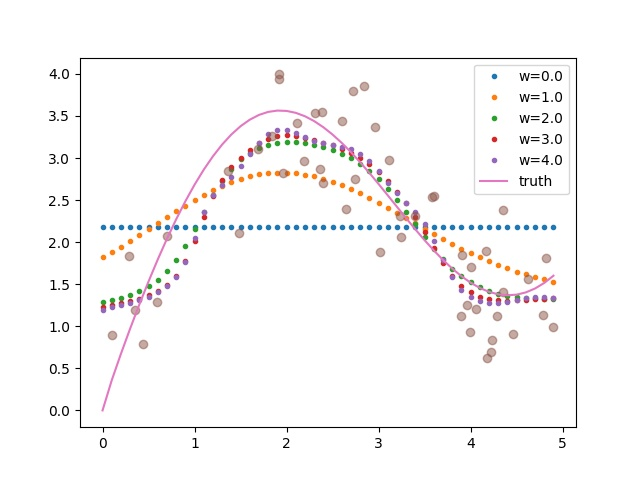1 Like

I am not sure I understand correctly, but wouldn’t in the case of x=x_i result in

sofmax(-1/2((x_i-x_i)*w)^2)

In which case the respective i_th prod would be zero?

Because x itself (key) does not have a label y (value): we are predicting its y

1 Like

# Inverse Kernel (Lenrek?)

Question 4 lead me onto a kernel potentially useful for the inverse purpose of most attention kernels.

## Kernel:

``````self.attention_weights = (
torch.abs((queries-keys)*self.w)
/torch.sum(torch.abs((queries - keys)*self.w), dim=(0))
)
``````

## Effect: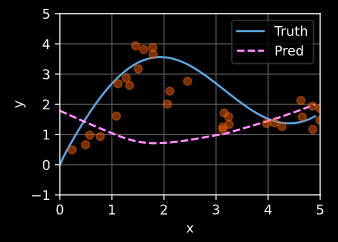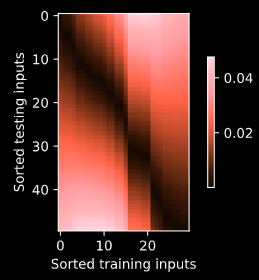## Description:

Does the opposite of what attention weights normally do:
Shifts focus AWAY from the closest neighbors.
Potentially useful for components focusing on long-range dependencies.

If you find it useful, please call this kerneL ‘Lenrek’.

1 Like
1 Like

conditon 1: Use all examples except itself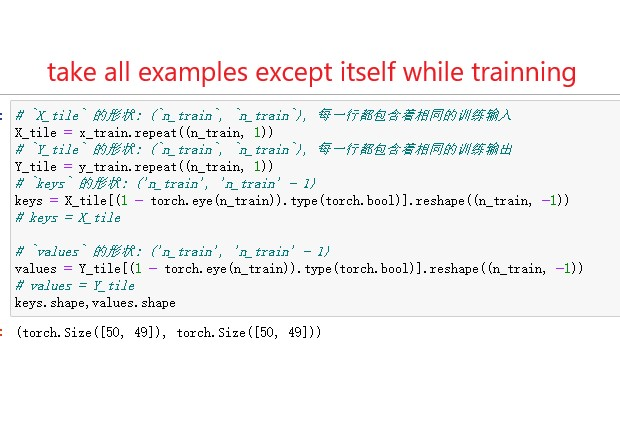1 Like
2 Likes

Having the learnable parameter w multiplying the distance between x and x_i after the squaring rather than before in the forward function seems to be smoother and less prone to overfitting, thus produces more desirable results :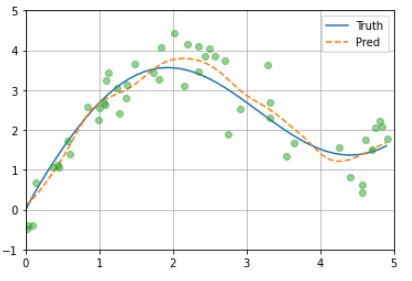What is the reasoning behind this behavior?

Q1: 1. Increase the number of training examples. Can you learn nonparametric Nadaraya-Watson kernel regression better?

With using 200 training and 200 testing examples, I found the performance of nonparametric Nadaraya-Watson kernel regression decreases with the increasing number of examples

Hi, for Nadarya-Watson Kernel regression, is it necessary that the inputs (X) are unidimensional? Can X_train be of the shape (n_train, d) where d > 1? If so how would we proceed with the problem.

@Raj_Sangani For `d >= 1`, you can define a distance function, e.g., Minkowski distance:

``````dist(x, z; p) = (x - z).abs().pow(p).sum().pow(1/p)
``````
1 Like